Course Content

0/1

0/3

0/5

0/3

0/2

0/4

0/2

0/1

0/5

5.2 Parabola 抛物线

1. Find the vertex, focus, directrix of the following parabola and sketch the graph.

(a)(b)(c)(d)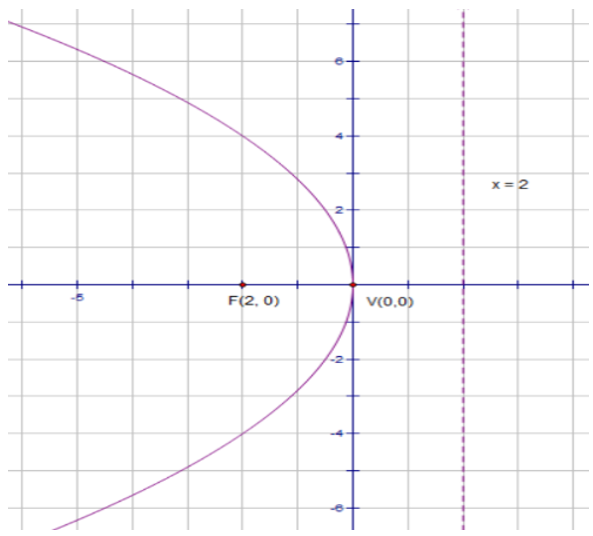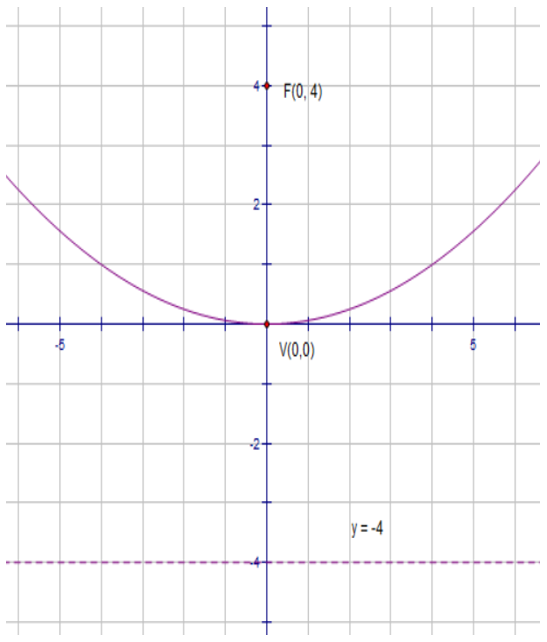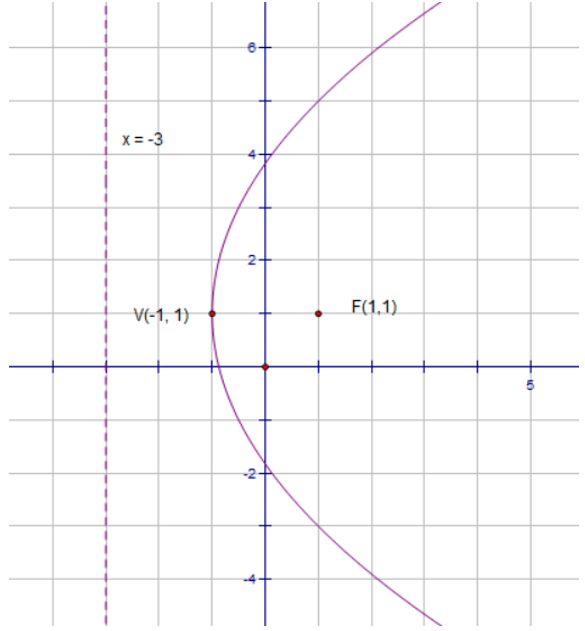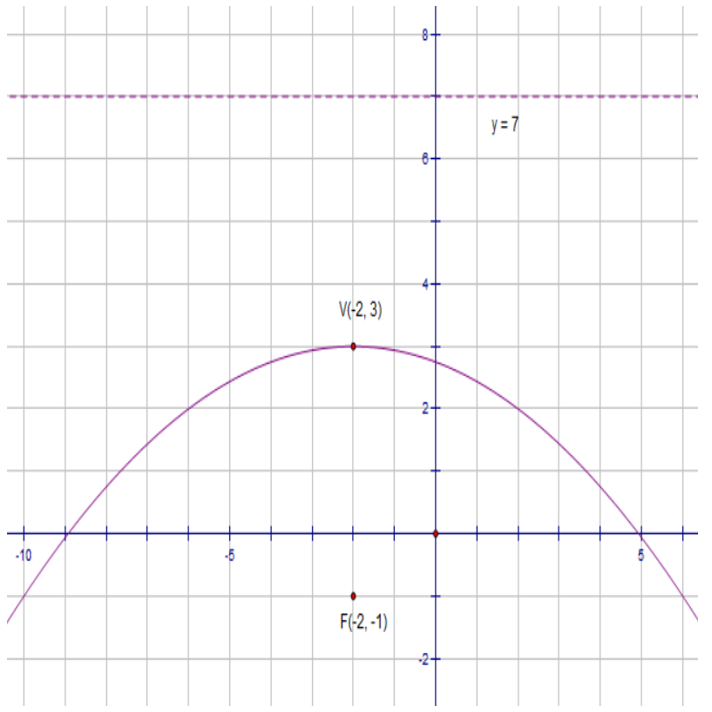2. Find the equation of the parabola with vertex at (5, -2) and focus at (5, -4)3. A parabola has its axis parallel to the y-axis, one end of its latus rectum is at (9, 6) and the vertex is at (5, 4). Find

(a) the length of the latus rectum

(b) the equation of the parabola

(a) 8

(b) x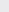– 10x – 8y + 57 = 0

4. Find the equation of a parabola where the vertex of the parabola is (-2, 3), the focus is on the line 4x – 3y + 5 = 0 and the symmetric axis is parallel to

(a) x – axis,
x-轴

(b) y – axis
y-坐标轴，

(a)(b)5. Find the equation of a parabola with vertex on the line y = 2x, symmetric axis parallel to the x-axis and passing through (1½, 1 ) and (3, 4),,,